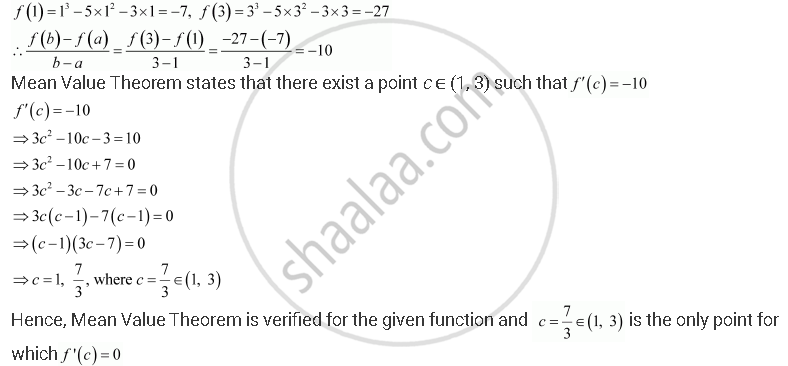Share
Notifications

View all notifications
Books Shortlist
Your shortlist is empty

# Solution for Verify Mean Value Theorem, If F (X) = X3 – 5x2 – 3x in the Interval [A, B], Where a = 1 and B = 3. Find All C ∈ (1, 3) for Which F ′(C) = 0. - CBSE (Science) Class 12 - Mathematics

Login
Create free account

Forgot password?

#### Question

Verify Mean Value Theorem, if f (x) = x3 – 5x2 – 3x in the interval [a, b], where a = 1 and b = 3. Find all c ∈ (1, 3) for which f ′(c) = 0.

#### Solution

The given function f is f (x) = x3 – 5x2 – 3x

f, being a polynomial function, is continuous in [1, 3] and is differentiable in (1, 3) whose derivative is 3x2 − 10x − 3.Is there an error in this question or solution?

#### APPEARS IN

NCERT Solution for Mathematics Textbook for Class 12 (2012 to Current)
Chapter 5: Continuity and Differentiability
Q: 5 | Page no. 186

#### Video TutorialsVIEW ALL 

Solution Verify Mean Value Theorem, If F (X) = X3 – 5x2 – 3x in the Interval [A, B], Where a = 1 and B = 3. Find All C ∈ (1, 3) for Which F ′(C) = 0. Concept: Mean Value Theorem.
S